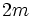# Converse of congruence condition on Sylow numbers for the prime two

## Statement

For any odd number$m$, there exists a finite group$G$ where the number of 2-Sylow subgroup (?)s of$G$ (i.e., the Sylow number (?) for the prime$2$) equals$m$.

## Proof

Further information: Dihedral group, subgroup structure of dihedral group

We take$G$ as the dihedral group$D_{2m}$ of degree$m$ and order$2m$. It is given by the presentation:$G := \langle a,x \mid a^m = x^2 = e, xax = a^{-1} \rangle$.

The two-element subgroup$\langle x \rangle$ is a 2-Sylow subgroup, and it is a self-normalizing subgroup. It has$m$ conjugates, given by$\langle a^r x \rangle$, with$0 \le r \le m - 1$.